# Physical Chemistry : Colligative Properties

## Example Questions

### Example Question #1 : Boiling Point

What is the boiling point of a 100mL solution of water after the addition of 29g of sodium chloride?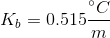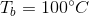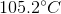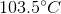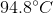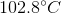Explanation:

We can use the boiling point elevation equation in order to find the new boiling point for the solution.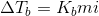The change in temperature will be equal to the boiling point elevation constant multiplied by the molality of the solution multiplied by the van't Hoff factor. The van't Hoff factor is a variable that incorporates how many ions the added solute will dissolve into when in solution. Sodium chloride will dissolve into two ions, so the van't Hoff factor is 2.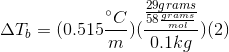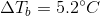This means that the boiling point of the water has been elevated by 5.2 degrees, meaning that the boiling point of the solution is 105.2 degrees celsius.

### Example Question #1 : Colligative Properties

When 20 grams of a solute is added to a liter of water, the freezing point becomes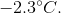What is the molar mass of the solute?

Assume that the solute does not make ions in solution.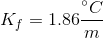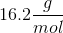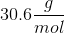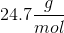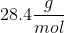Explanation:

We can use the freezing point depression equation in order to determine the molar mass of the unknown solute.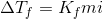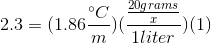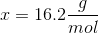### Example Question #1 : Colligative Properties

Which of the following is not an intensive property?

Temperature

Density

Pressure

Volume

Melting point